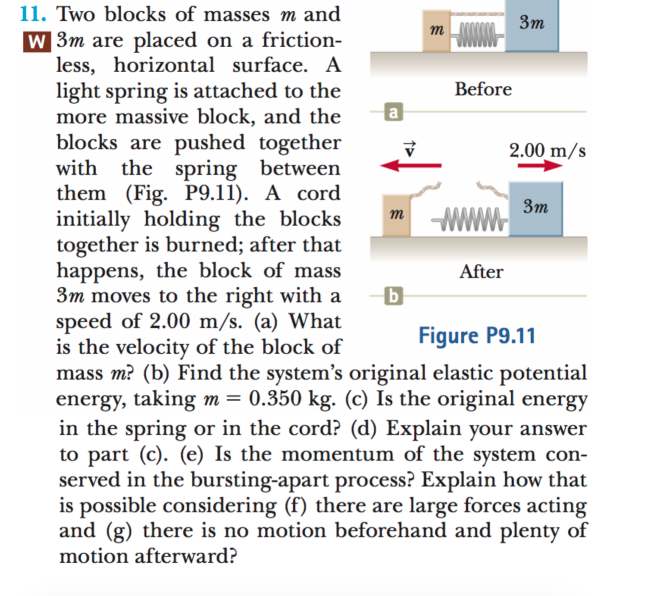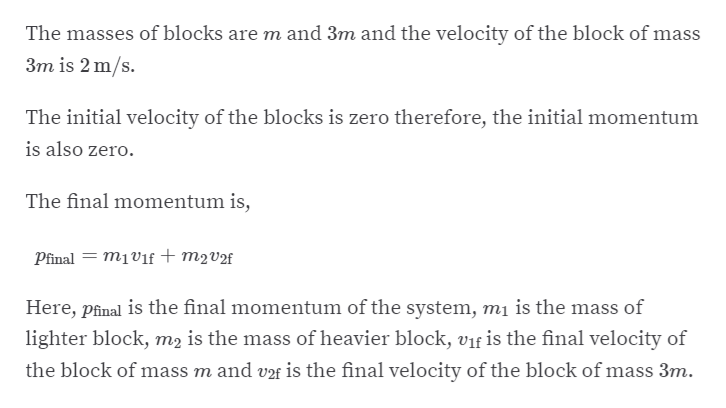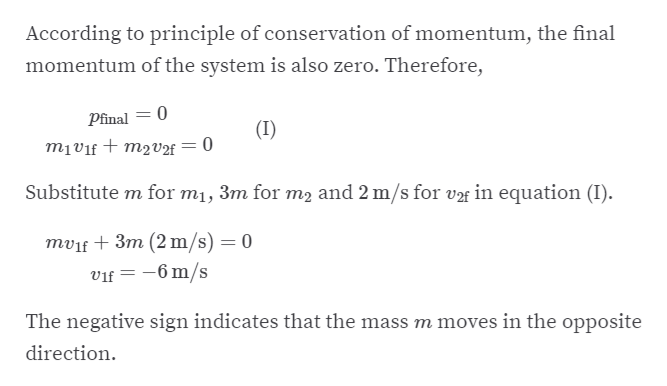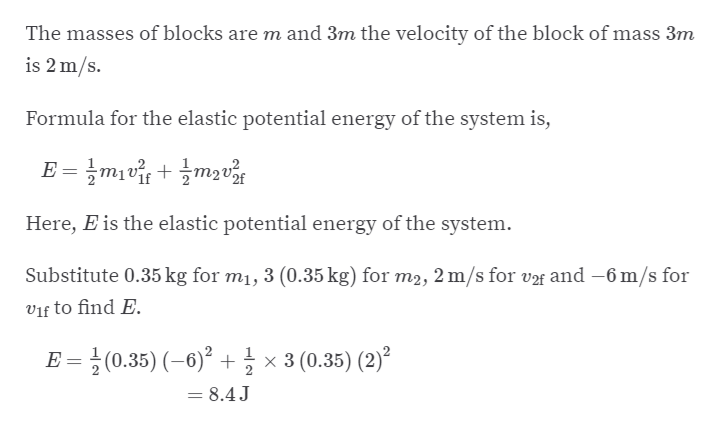# 11. Two blocks of masses m andЗттw 3m are placed on a friction-less, horizontal surface. Alight spring is attached to themore massive block, and theblocks are pushed togetherwith the spring betweenthem (Fig. P9.11). A cordinitially holding the blockstogether is burned; after thathappens, the block of mass3m moves to the right with aspeed of 2.00 m/s. (a) Whatis the velocity of the block ofmass m? (b) Find the system's original elastic potentialenergy, taking m= 0.350 kg. (c) Is the original energyin the spring or in the cord? (d) Explain your answerto part (c). (e) Is the momentum of the system con-served in the bursting-apart process? Explain how thatis possible considering (f) there are large forces actingand (g) there is no motion beforehand and plenty ofBefore2.00 m/sЗтWWWтAfterFigure P9.11motion afterward?

Question
2 viewshelp_outlineImage Transcriptionclose11. Two blocks of masses m and Зт т w 3m are placed on a friction- less, horizontal surface. A light spring is attached to the more massive block, and the blocks are pushed together with the spring between them (Fig. P9.11). A cord initially holding the blocks together is burned; after that happens, the block of mass 3m moves to the right with a speed of 2.00 m/s. (a) What is the velocity of the block of mass m? (b) Find the system's original elastic potential energy, taking m= 0.350 kg. (c) Is the original energy in the spring or in the cord? (d) Explain your answer to part (c). (e) Is the momentum of the system con- served in the bursting-apart process? Explain how that is possible considering (f) there are large forces acting and (g) there is no motion beforehand and plenty of Before 2.00 m/s Зт WWW т After Figure P9.11 motion afterward? fullscreen
check_circle

Step 1

(a)help_outlineImage TranscriptioncloseThe masses of blocks are m and 3m and the velocity of the block of mass 3m is 2 m/s. The initial velocity of the blocks is zero therefore, the initial momentum is also zero. The final momentum is, Pfinal = m1vif + m2v2f Here, pfinal is the final momentum of the system, mị is the mass of lighter block, m2 is the mass of heavier block, vif is the final velocity of the block of mass m and vf is the final velocity of the block of mass 3m. fullscreen
Step 2

Furtherhelp_outlineImage TranscriptioncloseAccording to principle of conservation of momentum, the final momentum of the system is also zero. Therefore, Pfinal = 0 (I) mịvif + m2v2f Substitute m for m1, 3m for m2 and 2 m/s for vf in equation (I). mvif + 3m (2 m/s) = 0 vif = -6 m/s The negative sign indicates that the mass m moves in the opposite direction. fullscreen
Step 3

(b)

...help_outlineImage TranscriptioncloseThe masses of blocks are m and 3m the velocity of the block of mass 3m is 2 m/s. Formula for the elastic potential energy of the system is, Eatu + atu = Here, E is the elastic potential energy of the system. Substitute 0.35 kg for m1, 3 (0.35 kg) for m2, 2 m/s for v2f and –6 m/s for vif to find E. E = } (0.35) (–6)² + × 3 (0.35) (2)² = 8.4J fullscreen

### Want to see the full answer?

See Solution

#### Want to see this answer and more?

Solutions are written by subject experts who are available 24/7. Questions are typically answered within 1 hour.*

See Solution
*Response times may vary by subject and question.
Tagged in

### Other